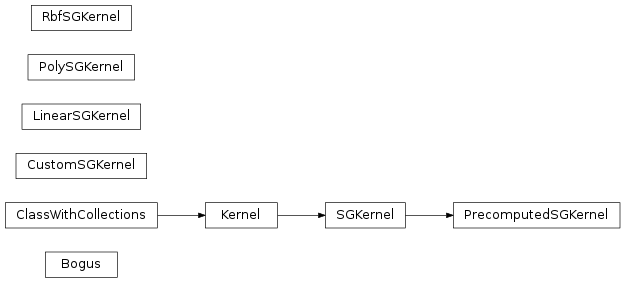# mvpa2.kernels.sg¶

PyMVPA shogun-based kernels

Provides interface to kernels defined in shogun toolbox. Commonly used kernels are provided with convenience classes: `LinearSGKernel`, `RbfSGKernel`, `PolySGKernel`. If you need to use some other shogun kernel, use `CustomSGKernel` to define one.Functions

 `exists`(dep[, force, raise_, issueWarning, ...]) Test whether a known dependency is installed on the system.

Classes

 `Bogus` `CustomSGKernel`(kernel_cls[, kernel_params]) Class which can wrap any Shogun kernel and it’s kernel parameters `Kernel`(\*args, \*\*kwargs) Abstract class which calculates a kernel function between datasets `LinearSGKernel`([normalizer_cls, normalizer_args]) A basic linear kernel computed via Shogun: K(a,b) = a*b.T `Parameter`(default[, constraints, ro, index, ...]) This class shall serve as a representation of a parameter. `PolySGKernel`(\*\*kwargs) Polynomial kernel: K(a,b) = (a*b.T + c)**degree `PrecomputedSGKernel`([matrix]) A kernel which is precomputed from a numpy array or a Shogun kernel `RbfSGKernel`(\*\*kwargs) Radial basis function: K(a,b) = exp(-||a-b||**2/sigma) `SGKernel`(\*args, \*\*kwargs) A Kernel object with internal representation in Shogun